Courses

# Friction Forces (Part -1) Civil Engineering (CE) Notes | EduRev

## Civil Engineering (CE) : Friction Forces (Part -1) Civil Engineering (CE) Notes | EduRev

The document Friction Forces (Part -1) Civil Engineering (CE) Notes | EduRev is a part of Civil Engineering (CE) category.
All you need of Civil Engineering (CE) at this link: Civil Engineering (CE)

2.5 Friction Forces

Friction forces act wherever two solids touch. It is a type of contact force – but rather more complicated than the contact forces we’ve dealt with so far.

It’s worth reviewing our earlier discussion of contact forces. When we first introduced contact forces, we said that the nature of the forces acting at a contact depends on three things:

(1) Whether the contact is lubricated, i.e. whether friction acts at the contact
(2) Whether there is significant rolling resistance at the contact
(3) Whether the contact is conformal, or nonconformal.

We have so far only discussed two types of contact (a) fully lubricated (frictionless) contacts; and (b) ideally rough (infinite friction) contacts.

Remember that for a frictionless contact, only one component of force acts on the two contacting solids, as shown in the picture on the left below. In contrast, for an ideally rough (infinite friction) contact, three components of force are present as indicated on the figure on the right.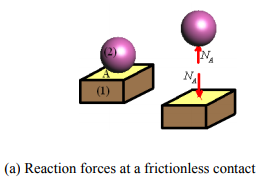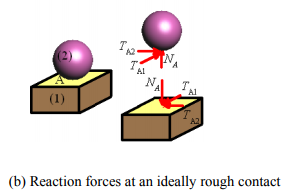All real surfaces lie somewhere between these two extremes. The contacting surfaces will experience both a normal and tangential force. The normal force must be repulsive, but can have an arbitrary magnitude. The tangential forces can act in any direction, but their magnitude is limited. If the tangential forces get too large, the two contacting surfaces will slip relative to each other.

This is why it’s easy to walk up a dry, rough slope, but very difficult to walk up an icy slope. The picture below helps understand how friction forces work. The picture shows the big MM walking up a slope with angleθ , and shows the forces acting on M and the slope. We can relate the normal and tangential forces acting at the contact to Mickey’s weight and the angleθ by doing a force balance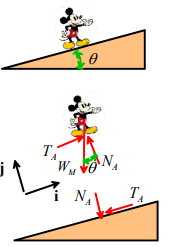Omitting the tedious details, we find that

TA = WM sin θ

NA = WM cos θ

Note that a tangential force sin TA =  WM = sin θ must act at the contact. If the tangential force gets too large, then Mickey will start to slip down the slope.

When we do engineering calculations involving friction forces, we always want to calculate the forces that will cause the two contacting surfaces to slip. Sometimes (e.g. when we design moving machinery) we are trying to calculate the forces that are needed to overcome friction and keep the parts moving. Sometimes (e.g. when we design self-locking joints) we need to check whether the contact can safely support tangential force without sliding.

2.5.1 Experimental measurement of friction forces

To do both these calculations, we need to know how to determine the critical tangential forces that cause contacting surfaces to slip. The critical force must be determined experimentally. Leonardo da Vinci was apparently the first person to do this – his experiments were repeated by Amontons and Coulomb about 100 years later. We now refer to the formulas that predict friction forces as Coulomb’s law or Amonton’s law (you can choose which you prefer!).

The experiment is conceptually very simple – it’s illustrated in the figure.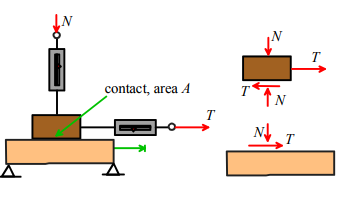We put two solids in contact, and push them together with a normal force N. We then try to slide the two solids relative to each other by applying a tangential force T. The forces could be measured by force transducers or spring scales. A simple equilibrium calculation shows that, as long as the weight of the components can be neglected, the contacting surfaces must be subject to a normal force N and a tangential force T.

In an experiment, a normal force would first be applied to the contact, and then the tangential force would be increased until the two surfaces start to slip. We could measure the critical tangential force as a function of N, the area of contact A, the materials and lubricants involved, the surface finish, and other variables such as temperature.

You can buy standard testing equipment for measuring friction forces – one configuration is virtually identical to the simple experiment described above – a picture is shown below. This instrument is used to measure friction between polymeric surfaces.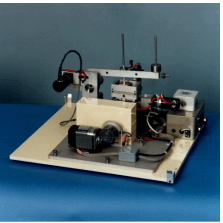There are many other techniques for measuring friction. One common configuration is the `pin on disk’ machine. Two examples are shown below. The picture on the left is from shows details of the pin and disk. The picture on the right, from shows a pin on disk experiment inside an environmental chamber. In this test, a pin is pressed with a controlled force onto the surface of a rotating disk. The force required to hold the pin stationary is measured.Another test configuration consists of two disks that are pressed into contact and then rotated with different speeds. The friction force can be deduced by measuring the torque required to keep the disks moving.

If you want to see a real friction experiment visit Professor Tullis’ lab at Brown (you don’t actually have to go there in person; he has a web site with very detailed descriptions of his lab) – he measures friction between rocks, to develop earthquake prediction models.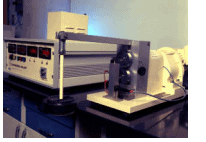A friction experiment must answer two questions:

(i) What is the critical tangential force that will cause the surfaces to start to slide? The force required to initiate sliding is known as the static friction force.
(ii) If the two surfaces do start to slip, what tangential force is required to keep them sliding? The force required to maintain steady sliding is referred to as the kinetic friction force.

We might guess that the critical force required to cause sliding could depend on

(i) The area of contact between the two surfaces
(ii) The magnitude of the normal force acting at the contact
(iii) Surface roughness
(iv) The nature of the crud on the two surfaces
(v) What the surfaces are made from

We might also guess that once the surfaces start to slide, the tangential force needed to maintain sliding will depend on the sliding velocity, in addition to the variables listed.

In fact, experiments show that

(i) The critical force required to initiate sliding between surfaces is independent of the area of contact. This is very weird. In fact, when Coulomb first presented this conclusion to the Academy Francaise, he was thrown out of the room, because the academy thought that the strength of the contact should increase in proportion to the contact area. We’ll discuss why it doesn’t below.

(ii) The critical force required to initiate sliding between two surfaces is proportional to the normal force. If the normal force is zero, the contact can’t support any tangential force. Doubling the normal force will double the critical tangential force that initiates slip.

(iii) Surface roughness has a very modest effect on friction. Doubling the surface roughness might cause only a few percent change in friction force.

(iv) The crud on the two surfaces has a big effect on friction. Even a little moisture on the surfaces can reduce friction by 20-30%. If there’s a thin layer of grease on the surfaces it can cut friction by a factor of 10. If the crud is removed, friction forces can be huge, and the two surfaces can seize together completely.

(v) Friction forces depend quite strongly on what the two surfaces are made from. Some materials like to bond with each other (metals generally bond well to other metals, for example) and so have high friction forces. Some materials (e.g. Teflon) don’t bond well to other materials. In this case friction forces will be smaller.

(vi) If the surfaces start to slide, the tangential force often (but not always) drops slightly. Thus, kinetic friction forces are often a little lower than static friction forces. Otherwise, kinetic friction forces behave just like static friction – they are independent of contact area, are proportional to the normal force, etc.

(vii) The kinetic friction force usually (but not always) decreases slightly as the sliding speed increases. Increasing sliding speed by a factor of 10 might drop the friction force by a few percent. Note that there are some exceptions to these rules. For example, friction forces acting on the tip of an atomic force microscope probe will behave completely differently (but you’ll have to read the scientific literature to find out how and why!). Also, rubbers don’t behave like most other materials. Friction forces between rubber and other materials don’t obey all the rules listed above.

2.5.2 Definition of friction coefficient: the Coulomb/Amonton friction law

A simple mathematical formula known as the Coulomb/Amonton friction law is used to describe the experimental observations listed in the preceding section.

Friction forces at 2D contacts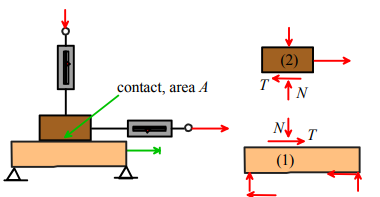Friction forces at a 2D contact are described by the following laws:

(i) If the two contacting surfaces do not slide, then

|T| < μN

(ii) The two surfaces will start to slip if

|T| = μN

(iii) If the two surfaces are sliding, then

T = ±μN

The sign in this formula must be selected so that T opposes the direction of slip.

In all these formulas, μ is called the `coefficient of friction’ for the two contacting materials. For most engineering contacts, 0 < μ < 1. Actual values are listed below.

Probably we need to explain statement (iii) in more detail. Why is there a ± ? Well, the picture shows the tangential force T acting to the right on body (1) and to the left on body (2). If (1) is stationary and (2) moves to the right, then this is the correct direction for the force and we’d use T = +μN . On the other hand, if (1) were stationary and (2) moved to the left, then we’d use T = -μN to make sure that the tangential force acts so as to oppose sliding.

Friction forces at 3D contacts

3D contacts are the same, but more complicated. The tangential force can have two components. To describe this mathematically, we introduce a basis {e1,e2,e3} with e1,e2 in the plane of the contact, and e3 normal to the contact. The tangential force T(1/2) exerted by body (1) on body (2) is then expressed as components in this basis

T(1/2) = T1e1 + T2e2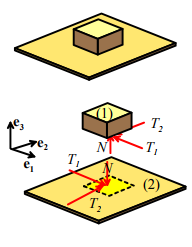(i) If the two contacting surfaces do not slide, then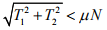(ii) The two surfaces will start to slip if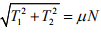(iii) If the two surfaces are sliding, then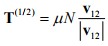where T(1/ 2) T denotes the tangential force exerted by body (1) on body (2), and v12 is the relative velocity of body (1) with respect to body (2) at the point of contact. The relative velocity can be computed from the velocities v1 and v2 of the two contacting solids, using the equation v12 = v1 - v2.

Offer running on EduRev: Apply code STAYHOME200 to get INR 200 off on our premium plan EduRev Infinity!

,

,

,

,

,

,

,

,

,

,

,

,

,

,

,

,

,

,

,

,

,

;